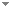Switch to: References

On the mathematical and foundational significance of the uncountable

Journal of Mathematical Logic 19 (1):1950001 (2019)

 Reverse Mathematics of Topology: Dimension, Paracompactness, and Splittings.Sam Sanders - 2020 - Notre Dame Journal of Formal Logic 61 (4):537-559.Reverse mathematics is a program in the foundations of mathematics founded by Friedman and developed extensively by Simpson and others. The aim of RM is to find the minimal axioms needed to prove a theorem of ordinary, that is, non-set-theoretic, mathematics. As suggested by the title, this paper deals with the study of the topological notions of dimension and paracompactness, inside Kohlenbach’s higher-order RM. As to splittings, there are some examples in RM of theorems A, B, C such that A (...) Direct download (3 more)     Export citationBookmarkSplittings and Disjunctions in Reverse Mathematics.Sam Sanders - 2020 - Notre Dame Journal of Formal Logic 61 (1):51-74.Reverse mathematics is a program in the foundations of mathematics founded by Friedman and developed extensively by Simpson and others. The aim of RM is to find the minimal axioms needed to prove a theorem of ordinary, that is, non-set-theoretic, mathematics. As suggested by the title, this paper deals with two RM-phenomena, namely, splittings and disjunctions. As to splittings, there are some examples in RM of theorems A, B, C such that A↔, that is, A can be split into two (...) Direct download (3 more)     Export citationBookmark2 citations Pincherle's Theorem in Reverse Mathematics and Computability Theory.Dag Normann & Sam Sanders - 2020 - Annals of Pure and Applied Logic 171 (5):102788.We study the logical and computational properties of basic theorems of uncountable mathematics, in particular Pincherle's theorem, published in 1882. This theorem states that a locally bounded function is bounded on certain domains, i.e. one of the first ‘local-to-global’ principles. It is well-known that such principles in analysis are intimately connected to (open-cover) compactness, but we nonetheless exhibit fundamental differences between compactness and Pincherle's theorem. For instance, the main question of Reverse Mathematics, namely which set existence axioms are necessary to (...) Direct download (2 more)     Export citationBookmark1 citation Reverse Mathematics and Parameter-Free Transfer.Benno van den Berg & Sam Sanders - 2019 - Annals of Pure and Applied Logic 170 (3):273-296. Direct download (2 more)     Export citationBookmark1 citation